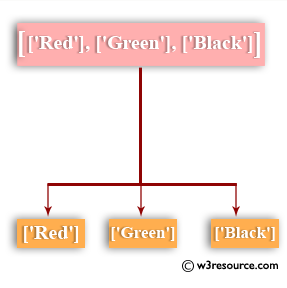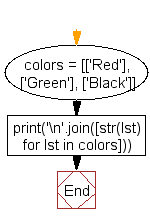﻿ Python: Print a nested lists using the print() function - w3resource# Python: Print a nested lists using the print() function

## Python List: Exercise - 48 with Solution

Write a Python program to print a nested lists (each list on a new line) using the print() function.Sample Solution:-

Python Code:

``````colors = [['Red'], ['Green'], ['Black']]
print('\n'.join([str(lst) for lst in colors]))
```
```

Sample Output:

```['Red']
['Green']
['Black']
```

Flowchart:## Visualize Python code execution:

The following tool visualize what the computer is doing step-by-step as it executes the said program:

Python Code Editor:

Have another way to solve this solution? Contribute your code (and comments) through Disqus.

What is the difficulty level of this exercise?

Test your Python skills with w3resource's quiz

﻿

## Python: Tips of the Day

Python: Debug With the Print() Function

```>>> for i in range(5):
...     print(i, end=', ' if i < 4 else '\n')
...
0, 1, 2, 3, 4
>>> for i in range(5):
...     print(f'{i} & {i*i}', end=', ' if i < 4 else '\n')
...
0 & 0, 1 & 1, 2 & 4, 3 & 9, 4 & 16
```